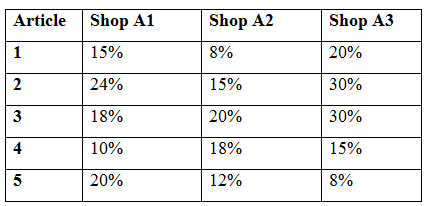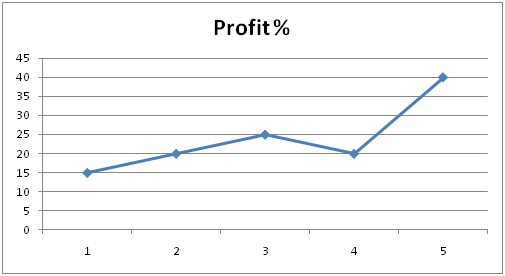# IBPS Clerk Mains Quantitative Aptitude Questions 2019 (Day-5) High Level New Pattern | With Video Explanation

Dear Aspirants, Quantitative Aptitude plays a crucial role in Banking and all other competitive exams. To enrich your preparation, here we have provided New Pattern Aptitude Questions for IBPS Clerk Mains. Candidates those who are going to appear in IBPS Clerk Mains can practice these questions daily and make your preparation effective.

Note: Dear IG ‘ians, Once Again IBPS Guide Team has brought you here with a New Innovative, to make you understand the questions more easier we have started providing Video Solutions for the questions. Practice here & click the link given below to check the video solutions of the same questions. Make use of it & thanks for your love.

[WpProQuiz 4646]

### Click Below for Video Solutions for these Questions

Directions (1-5): Study the following pie-chart and table carefully and answer the questions:

The Table below shows the discount % given by different shops A1, A2, A3 and the following line graph shows the profit % gained on the given articles by the given stores. It is given that Market Price of any article at every shop is same.1)

Quantity I: Find the marked price of an article in Shop A1, if the average cost price of an article 4 and 5 is Rs. 5550.

Quantity II: Find the marked price of an article in Shop A2, if the cost price of an article 2 Rs. 820 more than the cost price of an article 3

Quantity III: Find the marked price of an article in Shop A3, if the sum cost price of an article 1 and 4 is Rs. 19375

Which of the following should be placed in the blank spaces of the expression “Quantity I __ Quantity II ___ Quantity III” from left to right with respect to the above statements?

a) <, <

b) >, <

c) >, >

d) =, >

e) <, =

2) Find the CP of Article 4 if the average SP of this article in all the shops is Rs. 7710?

a) Rs 18504

b) Rs 19275

c) Rs 37014

d) Rs 27028

e) Rs 17765

3) What is the approximate difference between the CP of Article 3 of Shop A2 and Article 2 of Shop A3 if the MP is Rs. 2450?

a) Rs 235

b) Rs 255

c) Rs 217

d) Rs 139

e) Rs 282

4) If the CP of Article 2 of Shop A2 is greater than CP of Article 1 of Shop A3 by 350. What is the difference between the SP of Article 1 of Shop A2 and SP of Article 2 of A1?

a) Rs 4416

b) Rs 3818

c) Rs 4254

d) Rs 4602

e) Rs 3625

5) If Article 6 is introduced in Shop A1 with SP of Rs 4560 and if it equals the CP of Article 2 of same shop. What will be the approximate discount % on it?

a) 15 %

b) 20 %

c) 25 %

d) 37 %

e) 29%

Directions (6 – 10): What should come at the place of question mark in the following questions?

6) 1/7 x 1/5 x 4/9 x 3150 + 40% of 7800 =? – 540

a) 3700

b) 5600

c) 4400

d) 3200

e) None of these

7) 1/19 x 7638 + 1/26 x 2080 =? x 241

a) 3

b) 6

c) 4

d) 2

e) None of these

8) 343.55 + 533.43 – 4444.35 + 3353.54 =? – 333.33

a) 112.5

b) 114.5

c) 119.5

d) 110.5

e) None of these

9) √5776/4 – √4225 + √9604 x 5 =?

a) 444

b) 626

c) 740

d) 582

e) None of these

10) 80% of 24000 – 16% of 7200 =? – 15% of 2000

a) 12360

b) 18348

c) 20650

d) 16408

e) None of these

### Click Below for Video Solutions for these Questions

Direction (1-5) :

From Quantity I: Find the marked price of an article in Shop A1, if the average cost price of an article 4 and 5 is Rs. 5550.

Let us take the marked price of an article be M in shop A1,

SP of an article 4 = 90M/100

CP of an article 4 = 90M/100 *(100/120)

SP of an article 5 = 80M/100

CP of an article 5 = 80M/100 *(100/140)

Total CP of an article 4 and 5 in shop A1 = 5550 * 2 = Rs.11100

[90M/100*(100/120)] + [80M/100*(100/140)] = 11100

(90M/120 + 80M/140) = 11100

(630M+480M)/840 = 11100

1110M/840 = 11100

M = Rs. 8400

From Quantity II: Find the marked price of an article in Shop A2, if the cost price of an article 2 Rs. 820 more than the cost price of an article 3

Let us take the marked price of an article be M in shop A2

SP of an article 2 = 85M/100

CP of an article 2 = 85M/100 *(100/120)

SP of an article 3 = 80M/100

CP of an article 3 = 80M/100 *(100/125)

[85M/100 *(100/120)] – [80M/100 *(100/125)] = 820

85M/120 – 80M/125 = 820

2125M – 1920M = 820 *3000

205M = 820*3000

M = Rs.12000

From Quantity III: Find the marked price of an article in Shop A3, if the sum cost price of an article 1 and 4 is Rs. 19375

Let us take the marked price of an article be M in shop A3

SP of an article 1 = 80M/100

CP of an article 1= 80M/100 *(100/115)

SP of an article 4 = 85M/100

CP of an article 4 = 85M/100 *(100/120)

Total CP of an article 1 and 4 in shop A3 = 19375

[80M/100*(100/115)] + [85M/100*(100/120)] = 19375

80M/115 + 85M/120 = 19375

1920M + 1955M = 19375 * 2760

3875M = 19375 * 2760

M = Rs.13800

Hence, Quantity I < Quantity II < Quantity III

Let the MP of Article 4 be M,

SP of Shop A1 of Article 4 = 90M/100

SP of Shop A2 of Article 4 = 82M/100

SP of Shop A3 of Article 4 = 85M/100

Average of SP of Shop 1, 2 and 3 of Article

(i.e) ((90M/100) + (82M/100) + (85M/100))/3 = 7710

Solving the above equation, M = 9000

Total SP of Article 4= (90*9000/100) + (82*9000/100) + (85*9000/100)

= 257 * 9000/100

= 23130

Thus required CP = 23130*(100/120) = Rs. 19275

SP of Article 3 of shop A2 = (80*2450)/100

= Rs 1960

CP of Article 3 of Shop A2 = (1960 * 100)/125 = Rs. 1568

Again,

SP of Article 2 of Shop A3 = (70 * 2450)/100

= Rs 1715

CP of Article 2 of Shop A3 = (1715 * 100)/120 = Rs 1429.16

Difference between the CP of Article 3 of Shop A2 and Article 2 of Shop A2

= > 1568 – 1429.16 = Rs. 139

Let the MP be M,

CP of Article 2 of Shop A2 = (85M/100)*(100/120)

= 17M/24

CP of Article 1 of Shop A3 = (80M/100)* (100/115)

= 16M/23

As given in the Question,

17M/24 – 16M/23 = 350

M = 27600

Now, SP of Article 1 of Shop A2 = (92*27600)/100 = 25392

Again SP of Article 2 of Shop A1= (76*27600)/100

= 20976

Difference of SP of Article 1 of Shop A2 and SP of Article 2 of Shop A1

= 25392 – 20976 = Rs 4416

Let the MP be M and discount be x %,

CP of Article 2 of Shop A1 = (76M/100) * (100/120)

As per question,

(76M/100) *(100/120) = 4560

So, M = 7200

Again,

SP of Article 6 of Shop A1 = (100 – x) * 7200/100

(ie.,) (100 – x) * 7200/100 = 4560

100 – x = 4560*(100/7200)

100 – x = 63.33

x = 36.67 % = 37 %

Direction (6-10) :

1/7 x 1/5 x 4/9 x 3150 + 40% of 7800 = ? – 540

= > 40 + (40/100) x 7800 + 540 = ?

= > 40 + 3120 + 540 = ?

= > 3700 = ?

(1/19) x 7638 + (1/26) x 2080 =? x 241

= > 402 + 80 =? x 241

= > 482/241 =?

= > 2 =?

343.55 + 533.43 – 4444.35 + 3353.54 =? – 333.33

= > – 213.83 =? – 333.33

= > 333.33 – 213.83 =?

= > 119.5 =?

√5776/4 – √4225 + √9604 x 5 =?

= > 76/4 – 65 + 98 x 5 =?

= > 19 – 65 + 490 =?

= > 444 =?

80% of 24000 – 16% of 7200 =? – 15% of 2000

= > (80/100)*24000 – (16/100)*7200 =? – (15/100)*2000

= > 19200 – 1152 =? – 300

= > 19200 – 1152 + 300 =?

= > 18348 =?

Daily Practice Test Schedule | Good Luck

 Topic Daily Publishing Time Daily News Papers & Editorials 8.00 AM Current Affairs Quiz 9.00 AM Current Affairs Quiz (Hindi) 9.30 AM IBPS SO/NIACL AO Prelims – Reasoning 10.00 AM IBPS SO/NIACL AO Prelims – Reasoning (Hindi) 10.30 AM IBPS SO/NIACL AO Prelims – Quantitative Aptitude 11.00 AM IBPS SO/NIACL AO Prelims – Quantitative Aptitude (Hindi) 11.30 AM Vocabulary (Based on The Hindu) 12.00 PM IBPS SO/NIACL AO Prelims – English Language 1.00 PM SSC Practice Questions (Reasoning/Quantitative aptitude) 2.00 PM IBPS Clerk – GK Questions 3.00 PM SSC Practice Questions (English/General Knowledge) 4.00 PM Daily Current Affairs Updates 5.00 PM SBI PO/IBPS Clerk Mains – Reasoning 6.00 PM SBI PO/IBPS Clerk Mains – Quantitative Aptitude 7.00 PM SBI PO/IBPS Clerk Mains – English Language 8.00 PM